## Artigo

•Citado por SciELO
•Acessos

•Citado por Google
•Similares em SciELO
•Similares em Google

## versão impressa ISSN 0120-419X

### Integración - UIS vol.35 no.2 Bucaramanga jul./dez. 2017

#### http://dx.doi.org/10.18273/revint.v35n2-2017002

Artículos originales

On some asymptotic properties of classical Hermite polynomials modified by a rational factor

Sobre algunas propiedades asintóticas de polinomios de Hermite clásicos modificados por un factor racional

Luis Alejandro Molano Molano1

1Universidad Pedagógica y Tecnológica de Colombia. Escuela de Matemáticas y Estadística, Duitama, Colombia

Abstract

In this paper we study some asymptotic properties of the sequence of monic polynomials orthogonal with respect to the measure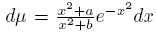, where a, b > 0 and ab. In this way we study the outer relative asymptotic with respect to the classical Hermite polynomials; besides, Mehler-Heine type formulas are analyzed.

MSC2010: 33C25, 33C45, 33C47, 42C05.

Key words: Asymptotics properties; perturbed Hermite polynomials; Christoffel and Geronimus perturbations

Resumen

En este artículo estudiamos algunas propiedades asintóticas de la sucesión de polinomios mónicos ortogonales con respecto a la medida, donde a, b > 0 y ab. En este sentido, estudiamos la asintótica relativa exterior con respecto a los polinomios clásicos de Hermite, además son analizadas fórmulas tipo Mehler-Heine.

Palabras-clave: Propiedades asintóticas; polinomios de Hermite perturbados; perturbaciones tipo Christoffel y Geronimus

1. Introduction

Let U and V be quasi-definite linear functionals and let {Pn } n∈N and {Rn } n∈N be their sequences of monic orthogonal polynomials (SMOP), respectively. (U, V ) is a coherent pair if there exists a sequence (an ), an ≠ 0, such that

This concept is introduced in  where it is studied its connection with polynomials orthogonal with respect to Sobolev inner products like

where μ 0 and μ 1 are positive Borel measures supported on an infinite subset I ⊆ R, with U and V as the associated functionals, respectively. In this way, among others, it is studied an algebraic connection between the Sobolev polynomials and the sequence {Rn } n∈N , in such a way that the coefficients of connection are independent of the degree; an algorithm is presented for to compute Fourier coefficients using as basis the Sobolev polynomials. Likewise, if U and V are symmetric, (U, V ) is a symmetric coherent pair if there exists a sequence (an ) , an ≠ 0, such that

In  all coherent pairs and symmetric coherent pairs are determined and it is shown that at least one of the functionals has to be classical (Hermite or Gegenbauer in the symmetric case); moreover, if ξ > 0, the symmetric coherent pair 0 = e x2 dx,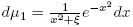is obtained. In connection with this particular case, in  the outer relative asymptotics of Sobolev polynomials orthogonal with respect to (1) is found; besides, in  Mehler-Heine type formulas are established with respect to rational modification of the Hermite polynomials. Under the same assumptions, (U, V ) is a symmetric (1, 1)−coherent pair if there exist sequences (an ) n∈N and (bn ) n∈N , bn ≠ 0, such that the respective SPOM satisfies

About this subject, in  is presented the algebraic relation between the Sobolev polynomials and the polynomials {Rn } n∈N ,; besides, the particular case where V is classical is studied, and the respective symmetric (1, 1)−coherent companion is found. In particular, we focus on the symmetric (1, 1)−coherent pair

and we will study the asymptotic behavior of the orthogonal polynomials associated with 1. Thus, the structure of this manuscript is as follows: In the section 2 we present some basic facts about of asymptotic behavior of Hermite orthogonal polynomials. In section 3 we present an algebraic connection between the Hermite polynomials and the SMOP associated with 1, as well as the asymptotic behavior of the respective connection coefficients. Finally, in section 4 some asymptotics properties are studied.

2. Preliminaries

From now on, and as it is usual, {Hn } n∈N will represent the sequence of monic Hermite polynomials, orthogonal with respect to the weight e x² on (−∞,∞). The classical Hermite linear functional will be denoted by H and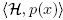will be the application of H on any polynomial p. The norm of the monic Hermite polynomials is defined as

On the other hand the sequence {Hn } n∈N is defined via the three terms recurrence relation

with the initial conditions H 0(x) = 1 and H −1(x) = 0. With respect to the asymptotic behavior of Hermite polynomials we present the next results.

Theorem 2.1 (See ).

uniformly on compact sets of C\R.

Theorem 2.2 (Mehler-Heine). (See ). For j ∈ Z fixed,

and

uniformly on compact sets of the complex plane, where Jα represents Bessel’s function of the first kind defined by

Theorem 2.3 (Mehler-Heine). (See ).

and

uniformly on compact subsets of C and uniformly on j ∈ N ∪ {0} .

Theorem 2.4 (See ). For j ∈ Z fixed,

holds uniformly on compact subsets of C\ [−√2,√2]. Here, φ (z) = z + √z 2 − 1 is the conformal mapping of C\ [−1, 1] onto the exterior of the closed unit disk.

If i = 0 and if p and q are non-negative integers such that n > p − 1, then

and as a consequence,

The above proves the next

Corollary 2.5. For j ∈ Z fixed, and non-negative integers p and q such that n > p − 1,

holds uniformly on compact subsets of C\ [−√2,√2].

The zeros of Hn are real, simples and symmetric; that is, for every n, Hn(t) = 0 is equivalent to Hn (−t) = 0. Let {xn ,k }[n/2] n=1 be the positive zeros of Hn in increasing order. It is well known that the zeros of Hn and Hn −1 are interlaced and for k fixed, xn ,k → 0 when n → ∞. Besides, given that Jα has a countably infinite set of real and positive zeros if α > −1, as a consequence of Mehler-Heine formulas and the Hurwitz’s theorem, if n → ∞ and k ≥ 1 then

and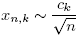, where ck > 0 and {jα ,k } n∈N are the zeros of Jα when α > −1.

On the other hand, let {Hn (a,b)} n∈N be the sequence of monic polynomials orthogonal with respect to the positive definite linear functional Ha b , defined as

where a, b > 0, and ab. As it is usual, let ║.║(a,b) be the induced norm. If c > 0, then {Hc n } will be the sequence of monic polynomials orthogonal with respect to the positive definite functional Hc defined by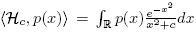, and ║.║ c the respective induced norm. On the algebraic connection between the sequence {Hc n } and the classical Hermite polynomials we get the next result.

Lemma 2.6 (See ). There exists a sequence of real numbers (σn ) such that

with

and

moreover,

With respect to asymptotic behavior and Mehler-Heine type formulas for the sequence {Hc n } n∈N , we get the next

Theorem 2.7 (See ).

and

both uniformly on compact subsets of C.

Let Lα be the classical Laguerre functional, α > −1, and let {Lα n (x)} be the respective SMOP. We present the next result about asymptotics behavior of ratios of Laguerre polynomials that will be necessary in our work. The proof can be see in .

Lemma 2.8. For x ∈ C\R+,

In this paper also will be important to deal with rational perturbations of the Lα and the asymptotic behavior of the associated SPOM. About this topic, in  is made an exhaustive study of asymptotic behavior of orthogonal polynomials associated to this kind of perturbations. Indeed, given c 1, c 2 < 0, let {Ln (α,c₁,c₂) (x)} be the SMOP associated to positive definite linear functional Lα c ₁,c defined on the space of polynomials as

and about the asymptotic behavior of the sequence {Ln (α,c₁,c₂) (x)},we get the next

Theorem 2.9 (See ).

a). Uniformly on compact subsets of C\[0,∞),

b). Uniformly on compact subsets of C,

where j ∈ N ∪ {0} .

c). (Plancherel-Rotach type exterior asymptotics).

uniformly on compact subsets of C\[0, 4], and uniformly on j ∈ N ∪ {0} .

3. Some basic results

Given that H is a symmetric linear functional, it is well known that there is a relation between the classical Laguerre and Hermite polynomials, namely

In this way, the next result is an extension of the above relations.

Lemma 3.1. For every n ∈ N,

Proof. Given that Ha b is symmetric, there exists an unique quasi-definite linear functional v, with {Pn } as the associated SPOM and such that H 2n (a,b) (x) = Pn (x 2) and H (a,b) 2n+1(x) = xPn (x 2) (see ), moreover,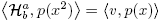.We will see that v = L −1/2 a,−b . Indeed, with the change of variable u = x 2 we get

On the other hand, from (22) we have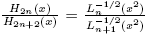, and given that (see (18))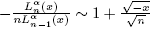, we get

in the same way, in the odd case we get

Then we have deduced the next

Lemma 3.2. For x ∈ C\R we get

By using of {Hb n } n∈N as a basis we get

where

then, cn ,j = 0, for j = 0, ..., n − 1, and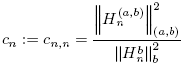. So we get

As a consequence of (12), we have

In order to obtain the behavior limit of the sequence (cn ), we choose x = ia in (25), and then for every n

By using (12) we get

In the even case,

From (12) we obtain

and by using of (18) we get

Given that H 2n+1(x) = xLn 1/2 (x 2), and following the same arguments in the odd case for the subsequence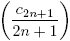, we get the next

Lemma 3.3.

4. Asymptotic Properties

We want to obtain formulas of the kind (4) associated to the ratios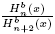and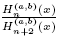. First, we will deduce the next

Lemma 4.1.

uniformly on compact sets of C\R.

Proof. From (12), we get that

and by using of (24) we get

then the result follows.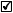In the above result we have obtained a formula of the type (4) for the sequence {Hb n } n∈N . Now we will get the same one for the sequence {Hn (a,b)} n∈N. Indeed, in the odd case and from (23) we get

as before, the even case is similar. Then, as a consequence of (18) and (19) we get the next

Proposition 4.2.

uniformly on compact subsets of C\R.

Moreover, from (23) we get

and as a consequence of (19) we obtain

Lemma 4.3. Uniformly on compact subsets of C\R,

On the other hand, scaling the variable, and by (23), we get

and

And from (20) we deduce

Lemma 4.4. Uniformly on compact subsets of C,

and

where j ∈ N ∪ {0} .

Finally, given that

and

as a consequence of (21) we have

Lemma 4.5 (Plancherel-Rotach type exterior asymptotics).

uniformly on compact subsets of C − [−2, 2], and uniformly on j ∈ N ∪ {0} .

In our search of information about of asymptotic behavior of the sequence {Hn (a,b)} n∈N, it is very useful to consider the sequence {(x 2 + a)Hn (a,b)} and the results obtained in the above lemmas. In this way, using (25) we obtain

then, the next proposition is a consequence of (27) and (28).

Proposition 4.6. The sequence {Hn (a,b)} satisfy

uniformly on compact sets of C\R.

Naturally, it is possible to obtain a similar result for the ratio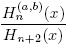. It is enough to consider (4) and (12) to obtain

uniformly on compact sets of C\R, and to use the above theorem for the expression

On the other hand, using recurrence relation (3) we get

and as a consequence,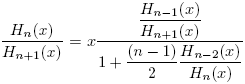. As before, for x ∈ C\R, and from (24), it follows

and as a consequence,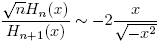. Then we get the next

Lemma 4.7.

uniformly on compact subsets of C\R.

Now, we will see the importance of above lemma in the following theorems.

Theorem 4.8.

uniformly on subsets compact of C\R.

Proof. From (26) we get

then, using (4) and (29) the result holds.Theorem 4.9 (Scaled Relative Asymptotics).

holds uniformly on compact subsets of C\ [−√2,√2].

Proof. Making the scaling x → √nx in (26), we have

Then, using (9) and (14) we obtain

Now we will deduce Mehler-Heine type formulas for the polynomials {Hn (a,b)}. Making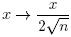in (25), for the even case we get

Then, using (16) and following a similar procedure in the odd case, we get the next

Proposition 4.10 (Mehler-Heine type formulas).

and

uniformly on compact subsets of the complex plane.

Acknowledgements

This work has been supported by Dirección de Investigaciones, Universidad Pedagógica y Tecnológica de Colombia, project code 1922.

References

 Abramowitz M. and Stegun I.A. (Eds.), Handbook of Mathematical Functions, 10th Edition, Dover, New York, 1972. [ Links ]

 Alfaro M., Moreno–Balcázar J.J., Pérez T.E., Piñar M.A. and Rezola M.L., “Asymptotics of Sobolev orthogonal polynomials for Hermite coherent pairs”, J. Comput. Appl. Math. 133 (2001), No. 1-2, 141–150. [ Links ]

 Chihara T.S., An Introduction to Orthogonal Polynomials, Gordon and Breach, New York, 1978. [ Links ]

 Delgado A.M. and Marcellán F., “On an extension of symmetric coherent pairs of orthogonal polynomial”, J. Comput. Appl. Math. 178 (2005), No. 1-2, 155–168. [ Links ]

 Dueñas H., Huertas E. andMarcellán F. “Asymptotic properties of Laguerre–Sobolev type orthogonal polynomials”, Numer. Algorithms 60 (2012), No. 1, 51–73. [ Links ]

 Fejzullahu B.Xh., “Asymptotics for orthogonal polynomials with respect to the Laguerre measure modified by a rational factor”, Acta Sci. Math. (Szeged) 77 (2011), No. 1-2, 73–85. [ Links ]

 Iserles A., Koch P.E., Norsett S.P. and Sanz-Serna J.M., “On polynomials orthogonal with respect to certain Sobolev inner products”, J. Approx. Theory 65 (1991), No. 2, 151–175. [ Links ]

 Meijer H.G., “Determination of all coherent pairs”, J. Approx. Theory 89 (1997), No. 3, 321–343. [ Links ]

 Moreno-Balcazar J.J., “Smallest zeros of some types of orthogonal polynomials: asymptotics”, J. Comput. Appl. Math. 179 (2005), No. 1-2, 289–301. [ Links ]

 Szego G., Orthogonal Polynomials, Amer. Math. Soc. Colloq. Publ., Vol. 23, Amer. Math. Soc., 4th Edition, Providence, 1975. [ Links ]

 Van Assche W., Asymptotics for orthogonal polynomials, Lecture Notes in Mathematics, Vol. 1265, Springer-Verlag, Berlin, 1987. [ Links ]

Received: June 13, 2017; Accepted: November 08, 2017This work is under License Creative Commons Attribution 4.0 International.